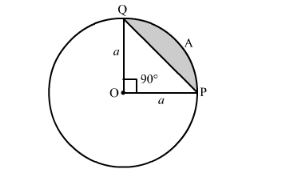# In the following figure, the area of the segment PAQ is

Question:

In the following figure, the area of the segment PAQ is

(a) $\frac{a^{2}}{4}(\pi+2)$

(b) $\frac{a^{2}}{4}(\pi-2)$

(c) $\frac{a^{2}}{4}(\pi-1)$

(d) $\frac{a^{2}}{4}(\pi+1)$Solution:

We have to find area of segment PAQ.

Area of the $\mathrm{PAQ}$ segment $=\left(\frac{\pi \theta}{360}-\sin \frac{\theta}{2} \cos \frac{\theta}{2}\right) r^{2}$

We know that $\theta=90^{\circ}$.

Substituting the values we get,

Area of the PAQ segment $=\left(\frac{\pi \times 90}{360}-\sin 45 \cos 45\right) a^{2}$

$\therefore$ Area of the PAQ segment $=\left(\frac{\pi}{4}-\sin 45 \cos 45\right) a^{2}$

Substituting $\sin 45=\frac{1}{\sqrt{2}}$ and $\cos 45=\frac{1}{\sqrt{2}}$ we get,

Area of the PAQ segment $=\left(\frac{\pi}{4}-\frac{1}{\sqrt{2}} \times \frac{1}{\sqrt{2}}\right) a^{2}$

$\therefore$ Area of the PAQ segment $=\left(\frac{\pi}{4}-\frac{1}{2}\right) a^{2}$

Now we will make the denominator same.

$\therefore$ Area of the PAQ segment $=\left(\frac{\pi}{4}-\frac{2}{4}\right) a^{2}$

$\therefore$ Area of the PAQ segment $=\left(\frac{\pi-2}{4}\right) a^{2}$

$\therefore$ Area of the PAQ segment $=(\pi-2) \frac{a^{2}}{4}$

$\therefore$ Area of the PAQ segment $=\frac{a^{2}}{4}(\pi-2)$

Therefore, area of the segment PAQ is $\frac{a^{2}}{4}(\pi-2)$.

Hence, the correct answer is option (b).